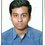Two identical blocks A and B each of mass M are placed on a long inclined plane (angle of inclination = θ ) with A higher up than B. The coefficients of friction between the plane and the blocks A and B are respectively µ A and µ B with tan θ > µ B > µ A. The two blocks are initially held fixed at a distance d apart. At t = 0 the two blocks are released from rest.

Find the time in which the two blocks collide?

My answer ; - $\large{t_{Collision} = \sqrt{\dfrac{2d \tan \theta ( \sin \theta - \mu_A \cos\theta)}{g (\sin \theta - \mu_B \cos\theta)}}}$

I am not sure with my answer, please all of us, lets discuss!Note by Md Zuhair
4 years, 3 months ago

This discussion board is a place to discuss our Daily Challenges and the math and science related to those challenges. Explanations are more than just a solution — they should explain the steps and thinking strategies that you used to obtain the solution. Comments should further the discussion of math and science.

When posting on Brilliant:

• Use the emojis to react to an explanation, whether you're congratulating a job well done , or just really confused .
• Ask specific questions about the challenge or the steps in somebody's explanation. Well-posed questions can add a lot to the discussion, but posting "I don't understand!" doesn't help anyone.
• Try to contribute something new to the discussion, whether it is an extension, generalization or other idea related to the challenge.

MarkdownAppears as
*italics* or _italics_ italics
**bold** or __bold__ bold
- bulleted- list
• bulleted
• list
1. numbered2. list
1. numbered
2. list
Note: you must add a full line of space before and after lists for them to show up correctly
paragraph 1paragraph 2

paragraph 1

paragraph 2

[example link](https://brilliant.org)example link
> This is a quote
This is a quote
    # I indented these lines
# 4 spaces, and now they show
# up as a code block.

print "hello world"
# I indented these lines
# 4 spaces, and now they show
# up as a code block.

print "hello world"
MathAppears as
Remember to wrap math in $$ ... $$ or $ ... $ to ensure proper formatting.
2 \times 3 $2 \times 3$
2^{34} $2^{34}$
a_{i-1} $a_{i-1}$
\frac{2}{3} $\frac{2}{3}$
\sqrt{2} $\sqrt{2}$
\sum_{i=1}^3 $\sum_{i=1}^3$
\sin \theta $\sin \theta$
\boxed{123} $\boxed{123}$

Sort by:

The two blocks will meet up after a time $t$ such that

$d + \dfrac{g}{2}(\sin(\theta) - \mu_{B}\cos(\theta)) = \dfrac{g}{2}(\sin(\theta) - \mu_{A}\cos(\theta))$,

which when solved for $t$ gives us $t_{c} = \sqrt{\dfrac{2d}{g\cos(\theta)(\mu_{B} - \mu_{A})}}$.

Suppose $\mu_{A} = \mu_{B}$. Then you wouldn't expect the two blocks to ever meet, but your formula would have them meeting after $\sqrt{\dfrac{2d\tan(\theta)}{g}}$ seconds, while mine would have $t_{C} \to \infty$ as expected.

- 4 years, 3 months ago

This seems to be a generalization of that "underkill" problem that was posted recently.

- 4 years, 3 months ago

Exactly. I found it a bit odd at first that $\cos(\theta)$ was in the denominator, since this would result in a finite $t_{c}$ for $\theta = 0$, which doesn't make sense, (although an infinite $t_{c}$ for $\theta = \pi/2$ does make sense as the two blocks would both be in free-fall so A would never catch up). But then I remembered that we require that

$\tan(\theta) \gt \mu_{B} \Longrightarrow \theta \gt \arccos\left(\dfrac{1}{\sqrt{1 + \mu_{B}^{2}}}\right)$.

- 4 years, 3 months ago

It would be interesting to consider this same problem with a curved surface and friction.

- 4 years, 3 months ago

Okay! So go on, Can you post one?

- 4 years, 3 months ago

I'll look into it

- 4 years, 3 months ago

- 4 years, 3 months ago

https://brilliant.org/problems/mechanics-overkill-2/?ref_id=1349100

- 4 years, 3 months ago

Thank you,

- 4 years, 3 months ago

One can generalise further : If the time at which they first meet is $t_{1}$, the subsequent times of meeting again (after collision) turns out to be odd multiples of $t_{1}$

- 4 years, 3 months ago

Hey! Whats your JEE Main score?

- 4 years, 3 months ago

around 290 :(

- 4 years, 3 months ago

Well, lets see your picture in newspaper!

- 4 years, 3 months ago

why?not at all!

my friends are expecting around 310-315

- 4 years, 3 months ago

Okay, leave that! You will surely qualify JEE Advanced with rank above 0.5k this year brother! I have seen your brain!

- 4 years, 3 months ago

well....

thank you!

- 4 years, 3 months ago

If you dont write advanced, ill delete my Brilliant account!

- 4 years, 3 months ago

So @Rohith M.Athreya are you giving either of ISI or CMI ?

- 4 years, 3 months ago

CMI yes

II no

- 4 years, 3 months ago

Thanks sir!

- 4 years, 3 months ago

Even I got the same answer as you sir. :)

@Md Zuhair From where did you get this problem?

- 4 years, 3 months ago

i did a very fine mistake! Its correct now!

- 4 years, 3 months ago

@Md Zuhair Yup, my answer matches with the one given by Brian Charlesworth and yes, its correct :-) ! Sorry yours was wrong :-( .

- 4 years, 3 months ago

Ya I got it now

- 4 years, 3 months ago

Let me tag some of you,

- 4 years, 3 months ago